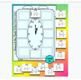# Multiplying and Dividing Fractions Partner Scavenger HuntSubject
Resource Type
File Type

PDF

(1 MB|6 pages)
Product Rating
Standards
• Product Description
• StandardsNEW

Students work in partners to multiply and divide fractions in this collaborative activity. Each partner gets a clock with 12 unique fraction problems. Each partner's clock problems are different but the answers are the same, encouraging collaboration and teamwork. If one student gets stuck, the other can help. If student answers differ, they work together to figure out the correct answer before moving on to the next problem.

Students paste their START slip at 12 o'clock, either multiply or divide its fractions and find the answer on the top of another slip. They then paste this next slip at 1 o'clock and solve its problem. Each answer students find will lead to the next pair of fractions to multiply or divide.

Students complete all 12 problems until they get back to 12 o'clock. If all fractions have been multiplied or divided correctly, the answer from the problem at 11 o'clock will be at the top of their START slip.

The activity is self-checking, providing students with instant feedback. The final product is a colorful example of their ability to work with fractions. Includes optional student answer sheets and answer key.

See more Partner Scavenger Hunts here.

You may also like:

Multiplying Fractions Math Pennant

Dividing Fractions using Models Task Cards Activity

Interpret and compute quotients of fractions, and solve word problems involving division of fractions by fractions, e.g., by using visual fraction models and equations to represent the problem. For example, create a story context for (2/3) ÷ (3/4) and use a visual fraction model to show the quotient; use the relationship between multiplication and division to explain that (2/3) ÷ (3/4) = 8/9 because 3/4 of 8/9 is 2/3. (In general, (𝘢/𝘣) ÷ (𝘤/𝘥) = 𝘢𝘥/𝘣𝘤.) How much chocolate will each person get if 3 people share 1/2 lb of chocolate equally? How many 3/4-cup servings are in 2/3 of a cup of yogurt? How wide is a rectangular strip of land with length 3/4 mi and area 1/2 square mi?
Apply and extend previous understandings of multiplication to multiply a fraction or whole number by a fraction.
Total Pages
6 pages
Included
Teaching Duration
N/A
Report this Resource to TpT
Reported resources will be reviewed by our team. Report this resource to let us know if this resource violates TpT’s content guidelines.